# Fuzzy Logic | Set 2 (Classical and Fuzzy Sets)

• Difficulty Level : Medium
• Last Updated : 24 Jan, 2022

Prerequisite : Fuzzy Logic | Introduction

In this post, we will discuss classical sets and fuzzy sets, their properties and operations that can be applied on them.
Set: A set is defined as a collection of objects, which share certain characteristics.

Classical set

1. Classical set is a collection of distinct objects. For example, a set of students passing grades.
2. Each individual entity in a set is called a member or an element of the set.
3. The classical set is defined in such a way that the universe of discourse is splitted into two groups members and non-members. Hence, In case classical sets, no partial membership exists.
4. Let A is a given set. The membership function can be use to define a set A is given by: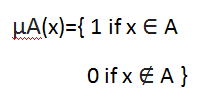1. Operations on classical sets: For two sets A and B and Universe X:
• Union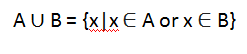• This operation is also called logical OR.
• Intersection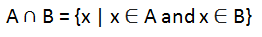• This operation is also called logical AND.
• Complement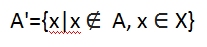• Difference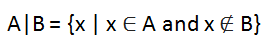1. Properties of classical sets: For two sets A and B and Universe X:
• Commutativity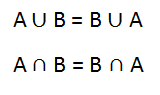• Associativity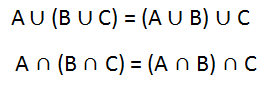• Distributivity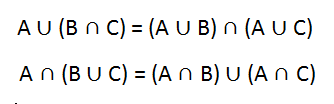• Idempotency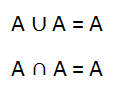• Identity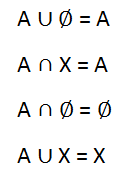• Transitivity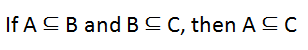Fuzzy set

1. Fuzzy set is a set having degrees of membership between 1 and 0. Fuzzy sets are represented with tilde character(~). For example, Number of cars following traffic signals at a particular time out of all cars present will have membership value between [0,1].
2. Partial membership exists when member of one fuzzy set can also be a part of other fuzzy sets in the same universe.
3. The degree of membership or truth is not same as probability, fuzzy truth represents membership in vaguely defined sets.
4. A fuzzy set A~ in the universe of discourse, U, can be defined as a set of ordered pairs and it is given by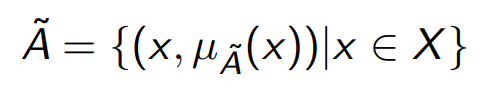1. When the universe of discourse, U, is discrete and finite, fuzzy set A~ is given by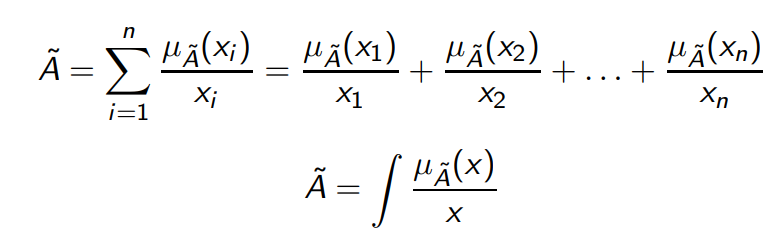1. Fuzzy sets also satisfy every property of classical sets.
2. Common Operations on fuzzy sets: Given two Fuzzy sets A~ and B~
• Union : Fuzzy set C~ is union of Fuzzy sets A~ and B~ :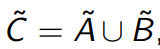•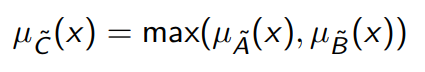• Intersection: Fuzzy set D~ is intersection of Fuzzy sets A~ and B~ :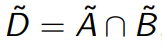•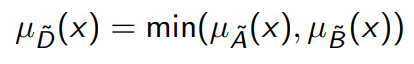• Complement: Fuzzy set E~ is complement of Fuzzy set A~ :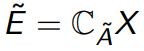•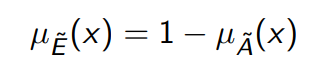1. Some other useful operations on Fuzzy set:
• Algebraic sum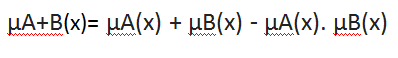• Algebraic product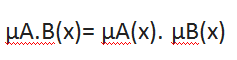• Bounded sum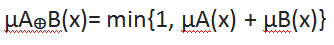• Bounded difference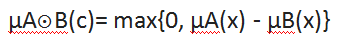My Personal Notes arrow_drop_up